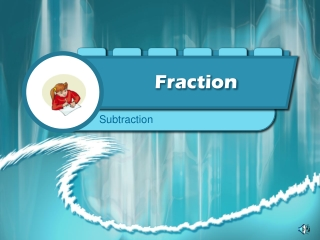DownloadDownload PresentationFraction

# Fraction

Télécharger la présentation## Fraction

- - - - - - - - - - - - - - - - - - - - - - - - - - - E N D - - - - - - - - - - - - - - - - - - - - - - - - - - -
##### Presentation Transcript

1. Fraction Subtraction

2. Simple Subtraction

3. Subtraction • Not to much different from addition. 1) Get a common Denominator 2) Subtract

4. Try one • ¾ - 2/5

5. Try one • 3 2/3 – 1 3/8

6. Subtraction With borrowing